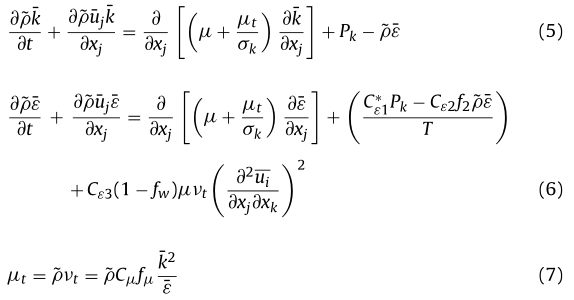# 新编译的湍流模型求解算例的时候epsilon方程发散

• 模型方程如下：我写的代码如下：

volScalarField G("RASModel::G", mut_*(gradU && dev(twoSymm(gradU))));

const volScalarField T(Ts());//新增

// Update epsilon and G at the wall
epsilon_.boundaryField().updateCoeffs();

// Dissipation equation
tmp<fvScalarMatrix> epsEqn
(
fvm::ddt(rho_, epsilon_)
+ fvm::div(phi_, epsilon_)
- fvm::laplacian(DepsilonEff(), epsilon_)
==
Ceps1_*G/T//C1_*G*epsilon_/k_
- fvm::SuSp((2.0/3.0)*Ceps1_*rho_*divU/T, k_)//- fvm::SuSp(((2.0/3.0)*C1_ + C3_)*rho_*divU, epsilon_)
- Ceps2_*rho_*f2()*epsilon_/T//fvm::Sp(Ceps2_*rho_*f2()/T, epsilon_)
- E
);

epsEqn().relax();

// No longer needed: matrix completes at the point of solution
// HJ, 17/Apr/2012
//     epsEqn().completeAssembly();

solve(epsEqn);
bound(epsilon_, epsilon0_);


报错如下：

pEqn.H is ok
min(p) [1 -1 -2 0 0 0 0] 3.99999e+06	max(p) [1 -1 -2 0 0 0 0] 4.15888e+06
piso is ok
BiCGStab:  Solving for fw, Initial residual = 1, Final residual = 0.000964975, No Iterations 69
new cannot satisfy memory request.
This does not necessarily mean you have run out of virtual memory.
It could be due to a stack violation caused by e.g. bad use of pointers or an out of date shared library
已放弃 (核心已转储)


麻烦大家看一下epsilon方程或者别的什么地方出现了问题

• new cannot satisfy memory request.
This does not necessarily mean you have run out of virtual memory.
It could be due to a stack violation caused by e.g. bad use of pointers or an out of date shared library


重新编译一下求解器试试？

• @东岳 我重新编译试过了，还是这个问题呢

CFD中文网 2016 - 2020 | 京ICP备15017992号-2